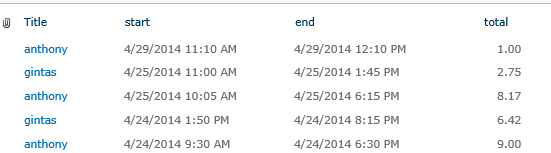# Average, Median, Min, Max functions

Applies to JungleDocs for SharePoint 2010-2019.

This article describes the formula syntax and usage of the Average, Median, Min and Max functions in JungleDocs.

## Description

The Average function calculates the average (mean) value from all the items specified in the record set by the specified field name.

The Median function calculates the median value from all the items specified in the record set by the specified field name.

The Min function returns the smallest of numbers from all the items specified in the record set by the specified field name.

The Max function returns the largest of numbers from all the items specified in the record set by the specified field name.

## Syntax

`Average(recordSet; fieldName)`

`Median(recordSet; fieldName)`

`Min(recordSet; fieldName)`

`Max(recordSet; fieldName)`

The functions' syntax has the following arguments:

• recordSet   Required. Any command that returns a list of items (e.g., GetView).
• fieldName   Required. The name of the column containing values that you want to calculate or compare.

## Example

Let's say you have the TimeSheet list with the AllItems view.Here's how you can get calculated values from the view:

`Average(GetView("TimeSheet"; "AllItems"); "total")`

Returns the average value from the total column.

`Median(GetView("TimeSheet"; "AllItems"); "total")`

Returns the median value from the total column.

`Min(GetView("TimeSheet"; "AllItems"); "total")`

Returns the lowest value from the total column.

`Max(GetView("TimeSheet"; "AllItems"); "total")`

Returns the highest value from the total column.